# MEAN

In statistics, mean has two related meanings:

• the arithmetic mean (and is distinguished from the geometric mean or harmonic mean).
• the expected value of a random variable, which is also called the population mean.

There are other statistical measures that use samples that some people confuse with averages – including ‘median’ and ‘mode’. Other simple statistical analyses use measures of spread, such as range, interquartile range, or standard deviation. For a real-valued random variable X, the mean is the expectation of X. Note that not every probability distribution has a defined mean (or variance); see the Cauchy distribution for an example.

For a data set, the mean is the sum of the values divided by the number of values. The mean of a set of numbers x1, x2, …, xn is typically denoted by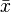, pronounced “x bar”. This mean is a type of arithmetic mean. If the data set were based on a series of observations obtained by sampling a statistical population, this mean is termed the “sample mean” () to distinguish it from the “population mean” (μ or μx). The mean is often quoted along with the standard deviation: the mean describes the central location of the data, and the standard deviation describes the spread. An alternative measure of dispersion is the mean deviation, equivalent to the average absolute deviation from the mean. It is less sensitive to outliers, but less mathematically tractable.

If a series of observations is sampled from a larger population (measuring the heights of a sample of adults drawn from the entire world population, for example), or from a probability distribution which gives the probabilities of each possible result, then the larger population or probability distribution can be used to construct a “population mean”, which is also the expected value for a sample drawn from this population or probability distribution. For a finite population, this would simply be the arithmetic mean of the given property for every member of the population. For a probability distribution, this would be a sum or integral over every possible value weighted by the probability of that value. It is a universal convention to represent the population mean by the symbol μ. In the case of a discrete probability distribution, the mean of a discrete random variable x is given by taking the product of each possible value of x and its probability P(x), and then adding all these products together, giving.

The sample mean may differ from the population mean, especially for small samples, but the law of large numbers dictates that the larger the size of the sample, the more likely it is that the sample mean will be close to the population mean.

As well as statistics, means are often used in geometry and analysis; a wide range of means have been developed for these purposes, which are not much used in statistics. These are listed below.

## Arithmetic mean (AM)

Main article: Arithmetic mean

The arithmetic mean is the “standard” average, often simply called the “mean”.The mean may often be confused with the median, mode or range. The mean is the arithmetic average of a set of values, or distribution; however, for skewed distributions, the mean is not necessarily the same as the middle value (median), or the most likely (mode). For example, mean income is skewed upwards by a small number of people with very large incomes, so that the majority have an income lower than the mean. By contrast, the median income is the level at which half the population is below and half is above. The mode income is the most likely income, and favors the larger number of people with lower incomes. The median or mode are often more intuitive measures of such data.

Nevertheless, many skewed distributions are best described by their mean – such as the exponential and Poisson distributions.

For example, the arithmetic mean of six values: 34, 27, 45, 55, 22, 34 is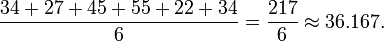### Geometric mean (GM)

Main article: Geometric mean

The geometric mean is an average that is useful for sets of positive numbers that are interpreted according to their product and not their sum (as is the case with the arithmetic mean) e.g. rates of growth.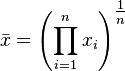For example, the geometric mean of six values: 34, 27, 45, 55, 22, 34 is: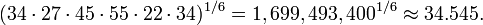### Harmonic mean (HM)

Main article: Harmonic mean

The harmonic mean is an average which is useful for sets of numbers which are defined in relation to some unit, for example speed (distance per unit of time).For example, the harmonic mean of the six values: 34, 27, 45, 55, 22, and 34 is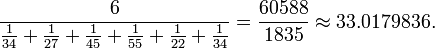### Relationship between AM, GM, and HM

Main article: Inequality of arithmetic and geometric means

AM, GM, and HM satisfy these inequalities: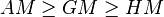Equality holds only when all the elements of the given sample are equal.

### Generalized means

#### Power mean

The generalized mean, also known as the power mean or Hölder mean, is an abstraction of the quadratic, arithmetic, geometric and harmonic means. It is defined for a set of n positive numbers xi byBy choosing the appropriate value for the parameter m we get all means: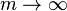maximum m = 2 quadratic mean m = 1 arithmetic mean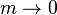geometric mean m = − 1 harmonic mean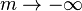minimum

#### ƒ-mean

This can be generalized further as the generalized f-meanand again a suitable choice of an invertible ƒ will giveharmonic mean, f(x) = xm power mean, f(x) = lnx geometric mean.

### Weighted arithmetic mean

The weighted arithmetic mean is used, if one wants to combine average values from samples of the same population with different sample sizes: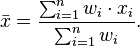The weights wi represent the bounds of the partial sample. In other applications they represent a measure for the reliability of the influence upon the mean by respective values.

### Truncated mean

Sometimes a set of numbers might contain outliers, i.e. a datum which is much lower or much higher than the others. Often, outliers are erroneous data caused by artifacts. In this case one can use a truncated mean. It involves discarding given parts of the data at the top or the bottom end, typically an equal amount at each end, and then taking the arithmetic mean of the remaining data. The number of values removed is indicated as a percentage of total number of values.

### Interquartile mean

The interquartile mean is a specific example of a truncated mean. It is simply the arithmetic mean after removing the lowest and the highest quarter of values.assuming the values have been ordered, so is simply a specific example of a weighted mean for a specific set of weights.

### Mean of a function

In calculus, and especially multivariable calculus, the mean of a function is loosely defined as the average value of the function over its domain. In one variable, the mean of a function f(x) over the interval (a,b) is defined by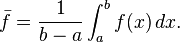Recall that a defining property of the average valueof finitely many numbersis that. In other words,is the constant value which when added to itself n times equals the result of adding the n terms of yi. By analogy, a defining property of the average value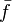of a function over the interval [a,b] is thatIn other words,is the constant value which when integrated over [a,b] equals the result of integrating f(x) over [a,b]. But by the second fundamental theorem of calculus, the integral of a constantis just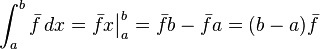See also the first mean value theorem for integration, which guarantees that if f is continuous then there exists a point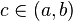such thatThe point f(c) is called the mean value of f(x) on [a,b]. So we writeand rearrange the preceding equation to get the above definition.

In several variables, the mean over a relatively compact domain U in a Euclidean space is defined by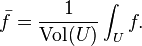This generalizes the arithmetic mean. On the other hand, it is also possible to generalize the geometric mean to functions by defining the geometric mean of f to be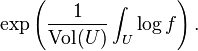More generally, in measure theory and probability theory either sort of mean plays an important role. In this context, Jensen’s inequality places sharp estimates on the relationship between these two different notions of the mean of a function.

There is also a harmonic average of functions and a quadratic average (or root mean square) of functions.

RELATED POST ::Welcome to my blog. My name is Nico. Admin of this blog. I am a student majoring in mathematics who dreams of becoming a professor of mathematics. I live in Kwadungan, Ngawi, East Java. Hopefully in all the posts I can make a good learning material to the intellectual life of the nation. After the read, leave a comment. I always accept criticism suggestion to build a better me again .. Thanks for visiting .. : mrgreen:

Posted on August 10, 2011, in STATISTIKA and tagged , , , , , , , . Bookmark the permalink. 2 Comments.

1.Cooper